# 一致性 Hash 算法jorden wang

http://www.open-open.com/lib/view/open1455374048042.html  一致性哈希算法的 Java 实现

# 引入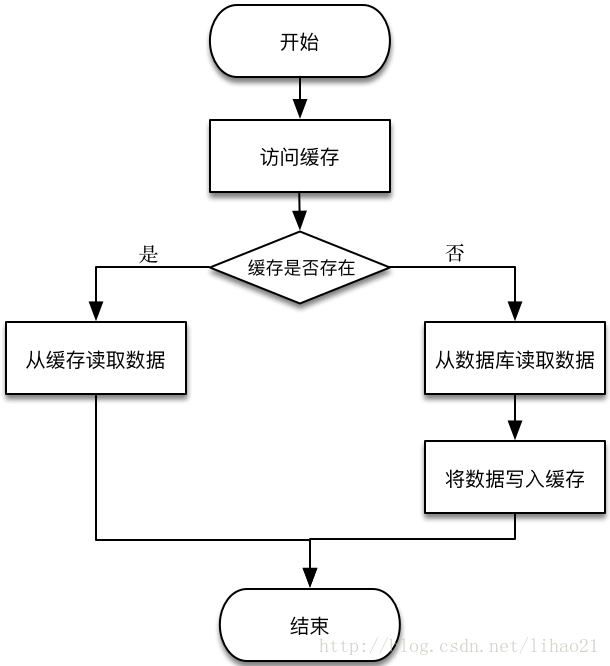m = hash(o) mod n ——式子 1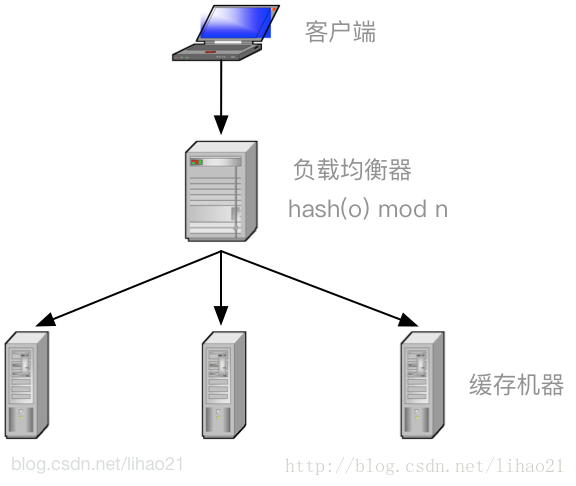m = hash(o) mod (n + 1) ——式子 2

m = hash(o) mod (n - 1) ——式子 3

# 一致性 Hash 环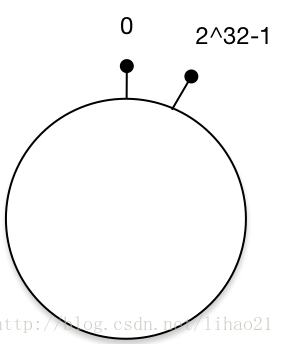# 将对象放置到 Hash 环

hash(o1) = m1

hash(o2) = m2

hash(o3) = m3

hash(o4) = m4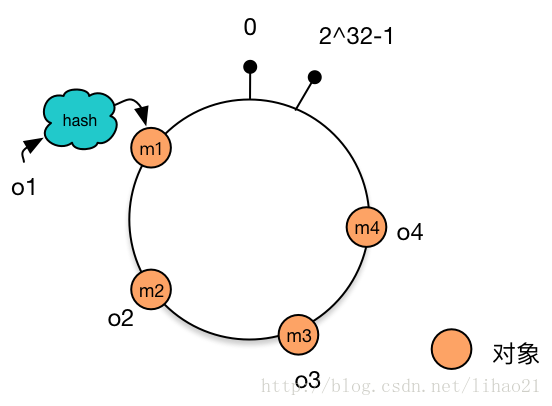# 将机器放置到 Hash 环

hash(c1) = t1

hash(c2) = t2

hash(c3) = t3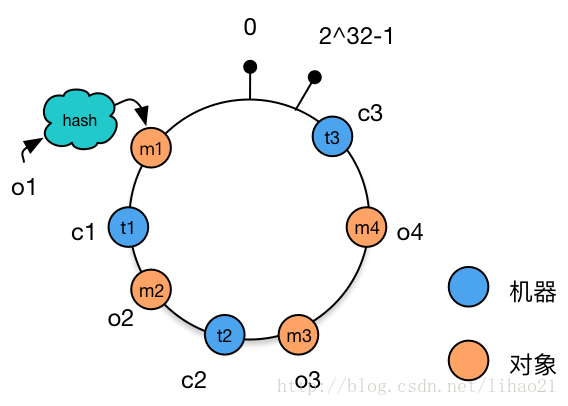# 为对象选择机器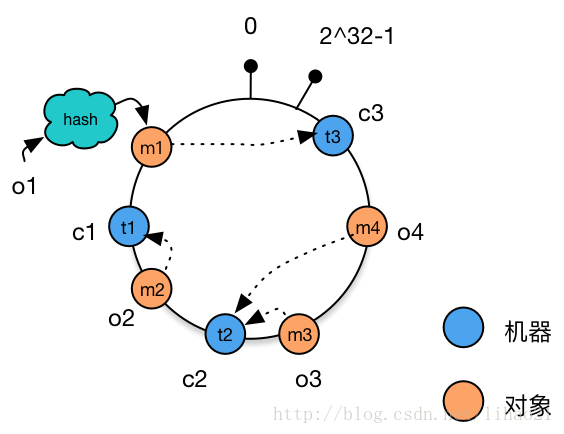# 处理机器增减的情况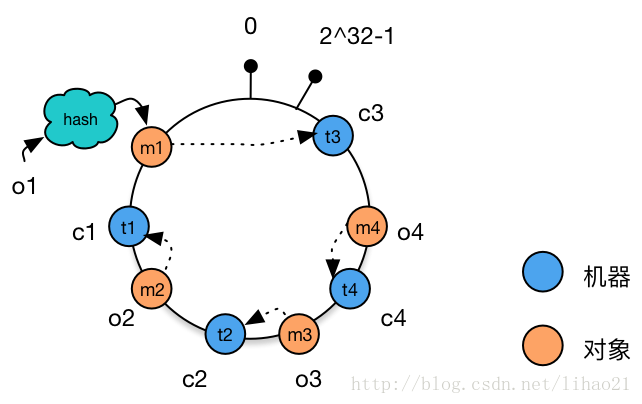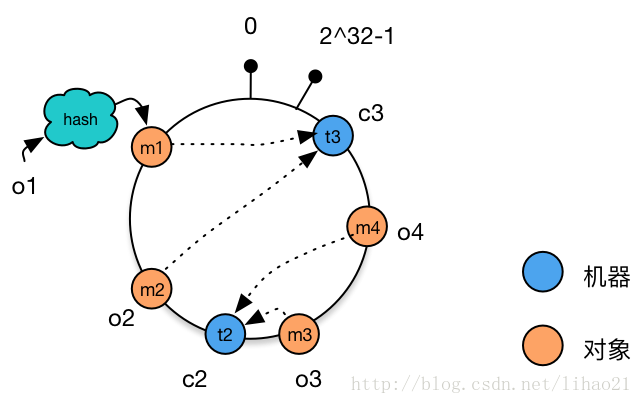# 虚拟节点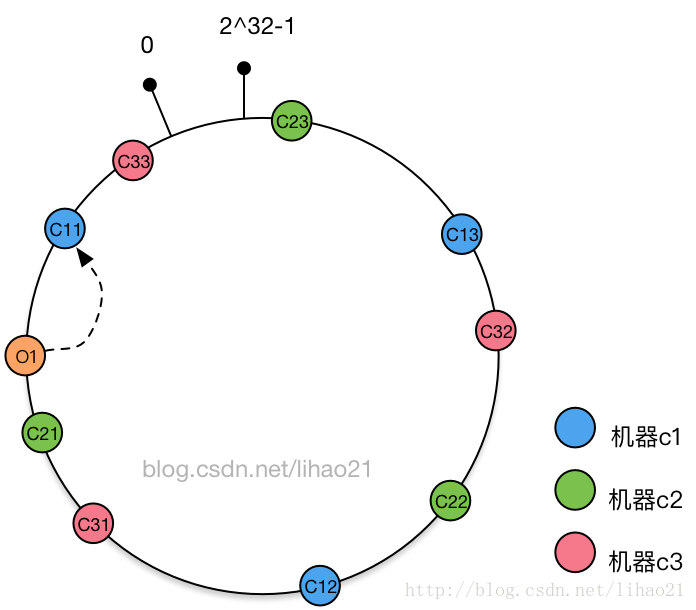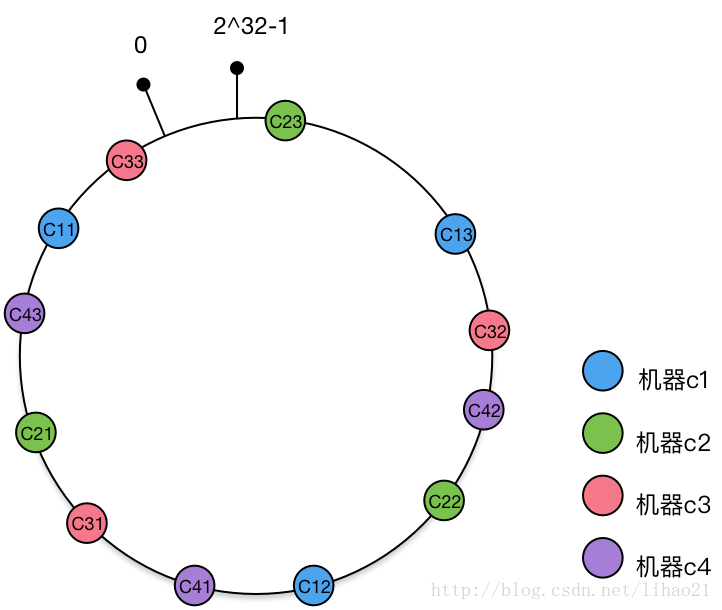## 二、一致性 hash 算法的 Java 实现

1、不带虚拟节点的

package hash; import java.util.SortedMap;import java.util.TreeMap; /** * 不带虚拟节点的一致性Hash算法 */public class ConsistentHashingWithoutVirtualNode { 	//待添加入Hash环的服务器列表	private static String[] servers = { "192.168.0.0:111", "192.168.0.1:111",			"192.168.0.2:111", "192.168.0.3:111", "192.168.0.4:111" }; 	//key表示服务器的hash值，value表示服务器	private static SortedMap<Integer, String> sortedMap = new TreeMap<Integer, String>(); 	//程序初始化，将所有的服务器放入sortedMap中	static {		for (int i=0; i<servers.length; i++) {			int hash = getHash(servers[i]);			System.out.println("[" + servers[i] + "]加入集合中, 其Hash值为" + hash);			sortedMap.put(hash, servers[i]);		}		System.out.println();	} 	//得到应当路由到的结点	private static String getServer(String key) {		//得到该key的hash值		int hash = getHash(key);		//得到大于该Hash值的所有Map		SortedMap<Integer, String> subMap = sortedMap.tailMap(hash);		if(subMap.isEmpty()){			//如果没有比该key的hash值大的，则从第一个node开始			Integer i = sortedMap.firstKey();			//返回对应的服务器			return sortedMap.get(i);		}else{			//第一个Key就是顺时针过去离node最近的那个结点			Integer i = subMap.firstKey();			//返回对应的服务器			return subMap.get(i);		}	}		//使用FNV1_32_HASH算法计算服务器的Hash值,这里不使用重写hashCode的方法，最终效果没区别	private static int getHash(String str) {		final int p = 16777619;		int hash = (int) 2166136261L;		for (int i = 0; i < str.length(); i++)			hash = (hash ^ str.charAt(i)) * p;		hash += hash << 13;		hash ^= hash >> 7;		hash += hash << 3;		hash ^= hash >> 17;		hash += hash << 5; 		// 如果算出来的值为负数则取其绝对值		if (hash < 0)			hash = Math.abs(hash);		return hash;		} 	public static void main(String[] args) {		String[] keys = {"太阳", "月亮", "星星"};		for(int i=0; i<keys.length; i++)			System.out.println("[" + keys[i] + "]的hash值为" + getHash(keys[i])					+ ", 被路由到结点[" + getServer(keys[i]) + "]");	}}

[192.168.0.0:111]加入集合中, 其 Hash 值为 575774686

[192.168.0.1:111]加入集合中, 其 Hash 值为 8518713

[192.168.0.2:111]加入集合中, 其 Hash 值为 1361847097

[192.168.0.3:111]加入集合中, 其 Hash 值为 1171828661

[192.168.0.4:111]加入集合中, 其 Hash 值为 1764547046

[太阳]的 hash 值为 1977106057, 被路由到结点[192.168.0.1:111]

[月亮]的 hash 值为 1132637661, 被路由到结点[192.168.0.3:111]

[星星]的 hash 值为 880019273, 被路由到结点[192.168.0.3:111]

2、带虚拟节点的

package hash; import java.util.LinkedList;import java.util.List;import java.util.SortedMap;import java.util.TreeMap; import org.apache.commons.lang.StringUtils; /**  * 带虚拟节点的一致性Hash算法  */ public class ConsistentHashingWithoutVirtualNode {      //待添加入Hash环的服务器列表     private static String[] servers = {"192.168.0.0:111", "192.168.0.1:111", "192.168.0.2:111",             "192.168.0.3:111", "192.168.0.4:111"};          //真实结点列表,考虑到服务器上线、下线的场景，即添加、删除的场景会比较频繁，这里使用LinkedList会更好     private static List<String> realNodes = new LinkedList<String>();          //虚拟节点，key表示虚拟节点的hash值，value表示虚拟节点的名称     private static SortedMap<Integer, String> virtualNodes = new TreeMap<Integer, String>();                  //虚拟节点的数目，这里写死，为了演示需要，一个真实结点对应5个虚拟节点     private static final int VIRTUAL_NODES = 5;          static{         //先把原始的服务器添加到真实结点列表中         for(int i=0; i<servers.length; i++)             realNodes.add(servers[i]);                  //再添加虚拟节点，遍历LinkedList使用foreach循环效率会比较高         for (String str : realNodes){             for(int i=0; i<VIRTUAL_NODES; i++){                 String virtualNodeName = str + "&&VN" + String.valueOf(i);                 int hash = getHash(virtualNodeName);                 System.out.println("虚拟节点[" + virtualNodeName + "]被添加, hash值为" + hash);                 virtualNodes.put(hash, virtualNodeName);             }         }         System.out.println();     }          //使用FNV1_32_HASH算法计算服务器的Hash值,这里不使用重写hashCode的方法，最终效果没区别     private static int getHash(String str){         final int p = 16777619;         int hash = (int)2166136261L;         for (int i = 0; i < str.length(); i++)             hash = (hash ^ str.charAt(i)) * p;         hash += hash << 13;         hash ^= hash >> 7;         hash += hash << 3;         hash ^= hash >> 17;         hash += hash << 5;                  // 如果算出来的值为负数则取其绝对值         if (hash < 0)             hash = Math.abs(hash);         return hash;     }          //得到应当路由到的结点     private static String getServer(String key){        //得到该key的hash值         int hash = getHash(key);         // 得到大于该Hash值的所有Map         SortedMap<Integer, String> subMap = virtualNodes.tailMap(hash);         String virtualNode;         if(subMap.isEmpty()){            //如果没有比该key的hash值大的，则从第一个node开始            Integer i = virtualNodes.firstKey();            //返回对应的服务器            virtualNode = virtualNodes.get(i);         }else{            //第一个Key就是顺时针过去离node最近的那个结点            Integer i = subMap.firstKey();            //返回对应的服务器            virtualNode = subMap.get(i);         }         //virtualNode虚拟节点名称要截取一下         if(StringUtils.isNotBlank(virtualNode)){             return virtualNode.substring(0, virtualNode.indexOf("&&"));         }         return null;     }          public static void main(String[] args){         String[] keys = {"太阳", "月亮", "星星"};         for(int i=0; i<keys.length; i++)             System.out.println("[" + keys[i] + "]的hash值为" +                     getHash(keys[i]) + ", 被路由到结点[" + getServer(keys[i]) + "]");     } }

[太阳]的 hash 值为 1977106057, 被路由到结点[192.168.0.2:111]

[月亮]的 hash 值为 1132637661, 被路由到结点[192.168.0.4:111]

[星星]的 hash 值为 880019273, 被路由到结点[192.168.0.3:111]## 评论